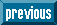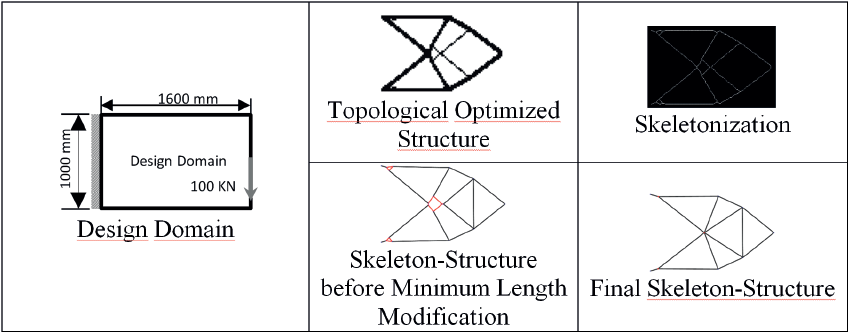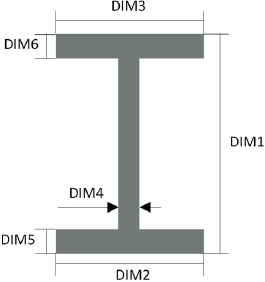## Indicators

•Cited by SciELO
•Access statistics

•Similars in SciELO

## Print version ISSN 1665-7381

### Ingenier. mecáni. tecnolog. desarroll vol.5 n.4 México Mar. 2016

Artículos

Adaptation of Topologic Optimized Structures Based on Skeletonization

a Centro de Diseño Mecánico e Innovación Tecnológica, Facultad de Ingeniería, Universidad Nacional Autónoma de México, Circuito Exterior S/N, Ciudad Universitaria, Distrito Federal, CP 04510, México. asahel_m@comunidad.unam.mx, fernvel@unam.mx, antonioz@unam.mx

Resumen:

Palabras clave: Diseño óptimo; diseño estructural; esqueletonización

Abstract:

The structural design based on optimization methods is very efficient in generating structures of minimum weight, maximum stiffness, etc.; nevertheless, it has the disadvantage of producing very complex geometries, whose manufacture is difficult or unfeasible. Therefore, these geometries have to be adapted to manufacturing processes, obtaining in this way feasible design solutions. During this adaptation process, several optimal characteristics are lost. In order to minimize this loss the authors propose an adaptation method, based on skeletonization, for topological solutions. The objective is to generate a manufacturable structure from a topological solution considering standard structural members. In this work, an algorithm to interpret and adapt the topological solution was developed. Skeletonization is applied to obtain a simpler representation of the optimal solution without losing its topology. The result is transformed in a set of straight lines joined by vertices. These lines are replaced by standard members, whose cross-section is parameterized. By applying size optimization, the dimensions of this cross-section are defined. As an example the method is applied to design an optimal short cantilever beam, showing a final structure completely manufacturable, whose topological characteristics are preserved.

Keywords: Optimal Design; Structural Design; Skeletonization

Introduction

Nowadays the use of optimization methods for solving structural problems has become very common because of their advantages versus conventional structural design methods. The lightest and stiffest structures can be designed by optimization methods, nevertheless they are very difficult to manufacture because these methods generate irregular and complex geometries, similar to those found in nature. Based on their experience, designers make several subjective decisions in order to simplify these geometries and make them manufacturable, at the expense of losing optimality. Some techniques have been developed to obtain regular geometries from optimized solutions without that losing. Chyi-Yeu & Shin-Hong  developed an algorithm based on an artificial neural network to identify geometrical patterns in optimized geometries; then these patterns are superpositioned to simplify the geometries. The main disadvantage is the limited number of regular geometries available. Ming-Hsiu & Yeh-Liang  and Yeh-Liang, Ming-Sho & Chuan-Tang  present automatic algorithms to interpret structures from a topological solution; with these methods the geometric irregularities are minimized, however the final geometry is still very irregular and complex, therefore quite difficult to manufacture.

The material distribution in geometries optimized by topological optimization is related with the structural functionality, which means material tends to be added and concentrated along areas where stress level is high, and eliminated along areas where stress level is low [4, 5]. The material distribution provides information to interpret the topological results as material paths.

Adaptation methodologies imply that designers have to make several decisions about which geometries are suitable to modify a geometry optimized by topological optimization. In the simplest approach, designers interpret that result as material paths along the design domain, as is shown in Figure 1; thereby the layout of material paths has a strong subjectivity. For that reason it is interesting to propose an automatic adaptation method, based in a computational algorithm, capable to generate material paths without subjectivity.Figure 1 Structural optimization of an electric delivery car . a) and b) show material paths adapted by a designer, c) Representation of the car with standard structural members. The subjectivity in the final result is quite high.

The adaptation method presented in this work is based on skeletonization, which is a morphological operation for image processing that transforms a binary image into its minimum representation (called skeleton) without losing its topological characteristics. Although there are several approaches for skeletonization process (morphological operators , curve evolution , level sets , among others), for all of them the skeleton of any geometry is a thin version of it, which is equidistant to its boundaries; in this way, topological properties of the geometry are emphasized instead of being lost. Once the skeleton is obtained, the vertices or intersections are identified. Subsequently, based on their connectivity, paths in the skeleton are replaced with straight lines. These lines represent structural members, whose cross-sectional dimensions are determined by size optimization. The final result is a structure that preserves optimal topological characteristics without subjectivity.

2. Method

In the following sections the method proposed is described. The set of steps is constituted for:

• Skeletonization.

• Vertices localization.

• Vertices connectivity and straight lines generation.

• Minimum length application.

2.1 Skeletonization

Skeletonization is an iterative process that consists in making a binary image thinner and thinner until its thickness is one pixel. The result is a set of connected paths that is known as skeleton, which preserves the base lines and connections that characterize the geometry, i.e. its topology. This operation is also known as medial axis transformation [10, 11] because all the lines are equidistant to the border of the geometry (Figure 2).

2.2 Vertices Localization

Once that skeleton is generated, the vertices or intersections are located. In this paper a vertex (V) is defined as a pixel where three or more paths converge. The skeleton is transformed in a matrix, where a white pixel is 1 and a black pixel is 0, therefore paths are represented by 1's in the matrix.

After, a submatrix of order 3x3 P11P12P13P21P22P23P31P32P33 is used to identify vertices. This submatrix is moved, inside the skeleton matrix, from the top left corner to the lower right corner, using unitary displacements from left to right and top to bottom. When the central position of this submatrix (P22) is located over a 1 value (white pixels), values of the adjacent positions are summed. If the sum is equal or higher than 3 the central pixel is marked as a vertex.

P11+P12+P13+P21+P23+P31+P32+P333 (1)

At the end of this process, all the inner vertices are found. In the other hand, there are two particular types of vertices that do not follow the described rule: 1) vertices at the start or the end of a path and 2) vertices with loads or constrains (boundary conditions). The Figure 3 shows a vertex type 1, for this vertex the sum of the adjacent positions is 1. For the type 2 the vertex must be identified explicitly.

If several paths concur at the same vertex, the described process generates a neighborhood of vertices (Figure 4). In order to obtain a single vertex in a neighborhood the following process is proposed, which is based on centroid calculation of a plane geometry.

First, the 3x3 submatrix is centered in each vertex (V) of the neighborhood and a local coordinate system is defined (Figure 5). The local coordinates of the centroid (X-,Y-) are calculated with the following expressions:

X-=iVxVi

and

Y-=iVyVi (2)

}

, where i goes from 1 to 3, Vx and Vy represent the number of vertices at a distance i in 𝑥 and 𝑦 direction respectively. In this way, the neighborhood of vertices is substituted for a new single vertex with coordinates X-,Y-. (Figure 6).

2.3 Vertices Connectivity and Straight Lines

The next step is to identify the vertices at the start and the end of each path, in order to replace these paths with straight lines. For this purpose the 3x3 submatrix is located in a vertex (start of the path) to identify adjacent pixels with value 1, these pixels indicate the direction of each path connected to the vertex. Then, the 3x3 submatrix is centered in one of the adjacent pixels with value 1. If the submatrix contains another vertex, this is the end of the path and its connectivity is defined by the start and end vertices; else the submatrix is centered in the next pixel with value 1 in the path until another vertex is reached. This process is repeated for each pixel with value 1 adjacent to the start vertex. (Figure 7).Figure 7 Connectivity between vertices V 1 and V 2 . Once the start and end vertices are found, the connectivity of a path is defined

When the connectivity of all the paths is known, these are replaced with straight lines. In this way the irregular topological result is transformed into a geometry topologically identical, but simpler than the original one. The structure obtained is called skeleton-structure.

2.4 Minimum Length Algorithm

The skeleton-structure might contain some very short members that hinder the manufacture process. For this reason the minimum length algorithm creates a submatrix that covers the vertices whose distance among them is less than a minimum value. This submatrix has the double size of the minimum length value, translated in pixels, in order to consider all the neighborhood of vertices that are very close; the minimum length must be specified explicitly.

The algorithm calculates a new vertex using the equation (2) but, given that the length of the submatrix is twice the minimum length, the distance of the vertices is recalculated until the vertices that do not fulfill the condition are vanished. This process is repeated until all the members which do not fulfill the minimum length are suppressed into a single vertex.

3. Case Study: the Short Cantilever Beam

As an application example, the short cantilever beam problem is addressed. The result of the topological structural optimization of the design domain and its adaptation based on the proposed method is shown in the Table 1. Evidently the final skeleton structure generated is topologically quite similar to the topological optimized structure.

Table 1 Adaptation of the topological optimized structure for the short beam problem.In this example the standard member in Figure 8 is selected (the selection of a feasible cross section is not considered in this paper, so it has to be a decision of the designer.); its cross-sectional dimensions are generated via size optimization. The objective is to define the optimal dimensions of the cross section to resist the boundary conditions (constrains and loads). The size optimization process is performed using OptiStruct from the Altair HyperWorks® software.Figure 8 Parametric cross-sectional dimensions of the standard member.

All the straight lines are meshed with 1D finite elements as is shown in Figure 9. The cross-sectional dimensions are considered as design variables, whose initial values are presented in Table 2. The objective function is set to minimize the compliance for a mass percentage of 25%. Structural steel ASTM A36 is selected as the material of the structure.

Table 2 Cross-sectional initial dimensions

Dimension Initial Value mm
DIM1 127
DIM2 76
DIM3 76
DIM4 5.4
DIM5 8.3
DIM6 8.3

The results of the optimal solution are shown in Table 3. In order to select a standard member, the S75x8.5 member is chosen because its dimensions are the closest to the optimal results.

Table 3 Optimal cross-sectional and S75X8.5 dimensions.

Dimension Optimum Values mm S75x8.5 Shape mm
DIM1 78.82 76
DIM2 36.45 59
DIM3 36.45 59
DIM4 2.459 4.3
DIM5 3.963 6.6
DIM6 3.963 6.6

FEA is applied to the structure considering the S75x8.5 standard member to evaluate the performance of the structure . In Figure 10 the maximum stress in each bar is shown. The Safety Factor (SF) of the structure is 1.2.

Finally, in order to complete the structural design, four different options for connections are presented, considering that all the joints are bolted or welded (Figure 11).Figure 11 Different options for joints in the final design.

4. Other Cases Study

The following Table shows the results of applying the proposed algorithm to other two classical optimization problems: Michell's structure and a bridge structure . In both cases the efficient performance of the algorithm is proved. Once again the topology of the optimal structure is preserved, without the loss of optimality associated with subjective decisions.

5. Conclusions

The adaptation algorithm presented is useful for topologically optimized structures because it transforms a non-manufacturable structure into a totally manufacturable one. Once the algorithm is applied, the skeleton-structure obtained preserves, without subjectivity, the topology of the optimal solution.

The minimum length algorithm allows the designer to control the existence of short members, which hinder manufacture, without affecting the topology significantly. Size optimization allows to select an appropriate standard member, which ensures withstand the boundary conditions.

In the first case study, the purpose of showing four different options for connections is to emphasize that even though the presented algorithm generates a unique solution for a specific structure, the problem of automatically generating connections has not been solved yet.

Adaptation for manufacturing of topologically optimized structures can be a very difficult issue for a person, because it strongly depends on that person's experience. The method presented in this work eliminates that dependence, which means a very convenient tool for structural design. In this sense, an optimized structure can preserve its optimality after manufacturing.

6. Acknowledgements

The financial support from DGAPA (PAPIIT-IN113315 and PAPIME-PE104515 projects) of Universidad Nacional Autónoma de México is highly appreciated.

7. References

 Chyi-Yeu, L., & Shin-Hong, L. (2005). Artificial neural network based hole image interpretation techniques for integrated topology and shape optimization. Computer methods in applied mechanics and engineering(194), 3817-3837. [ Links ]

 Ming-Hsiu, H., & Yeh-Liang, H. (2005). Interpreting three-dimensional structural topology optimization results. Computers & Structures(83), 327-337. [ Links ]

 Yeh-Liang, H., Ming-Sho, H., & Chuan-Tang, C. (2001). Interpreting results from topology optimization using density contours. Computers & Structures(79), 1049-1058. [ Links ]

 Xie, M.Y. and Steven G. P. (1997). Evolutionary structural optimization, Springer. [ Links ]

 Huang X. and Xie Y.M. (2010). Evolutionary Topology Optimization of Continuum Structures: Methods and Applications, Wiley. [ Links ]

 Camacho, F. A. (2011). Grupo de diseño mecánico óptimo : diseño conceptual de un vehículo eléctrico de reparto, modelado como un monocasco. Tesis. Ciudad Universitaria, Mexico D.F.: UNAM, Facultad de Ingeniería. [ Links ]

 Gonzalez, R.C. and Woods, R.E. (2008). Digital Image Processing. New Jersey. Pearson-Prentice Hall. [ Links ]

 Bai, X., Latecki, L.J. and Liu, W.Y. (2007). Skeleton Pruning by Contour Partitioning with Discrete Curve Evolution. IEEE Transactions on Pattern Analysis and Machine Intelligence, Vol(29), 1-14. [ Links ]

 Sethian, J.A. (1999). Level set methods and fast marching methods, 2nd ed. New York. Cambridge University Press. [ Links ]

 Prinz, F.B. and Chern, J.H. (1988). Geometric abstractions using medial axis transformation, Research Showcase. Carnegie Mellon University. [ Links ]

 Andrés Solís Montero and Jochen Lang (2012). Skeleton pruning by contour approximation and the integer medial axis transform. Computers & Graphics, Vol(36), 477-487. [ Links ]

 Bahtti, M.A. (2005). Fundamental finite element analysis and applications: with Mathematica and Matlab computations. New Jersey, John Wiley & Sons Inc. [ Links ]

 Querin, O.M. (1997). Evolutionary Structural Optimization: strees based formulation and implementation, Ph. D. thesis, Department of Aeronautical Engineering, University of Sydney, Australia. [ Links ]

Received: October 23, 2015; Accepted: March 15, 2016Este es un artículo publicado en acceso abierto bajo una licencia Creative Commons